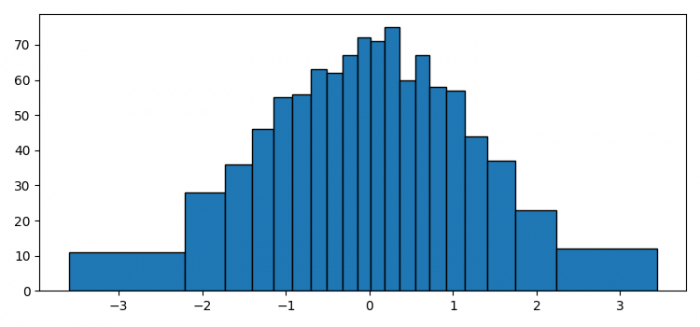# How to make a histogram with bins of equal area in Matplotlib?

To make a histogram with bins of equal area in matplotlib, we can take the following steps −

## Steps

• Set the figure size and adjust the padding between and around the subplots.

• Create random data points using numpy.

• Plot a histogram with equal_area method that makes an equal area of the patches.

• To display the figure, use show() method.

## Example

import numpy as np
from matplotlib import pyplot as plt

plt.rcParams["figure.figsize"] = [7.50, 3.50]
plt.rcParams["figure.autolayout"] = True

def equal_area(x, nbin):
pow = 0.5
dx = np.diff(np.sort(x))
tmp = np.cumsum(dx ** pow)
tmp = np.pad(tmp, (1, 0), 'constant')
return np.interp(np.linspace(0, tmp.max(), nbin + 1), tmp, np.sort(x))

x = np.random.randn(1000)

n, bins, patches = plt.hist(x, equal_area(x, 20), edgecolor='black')

plt.show()

## Output

It will produce the following output −